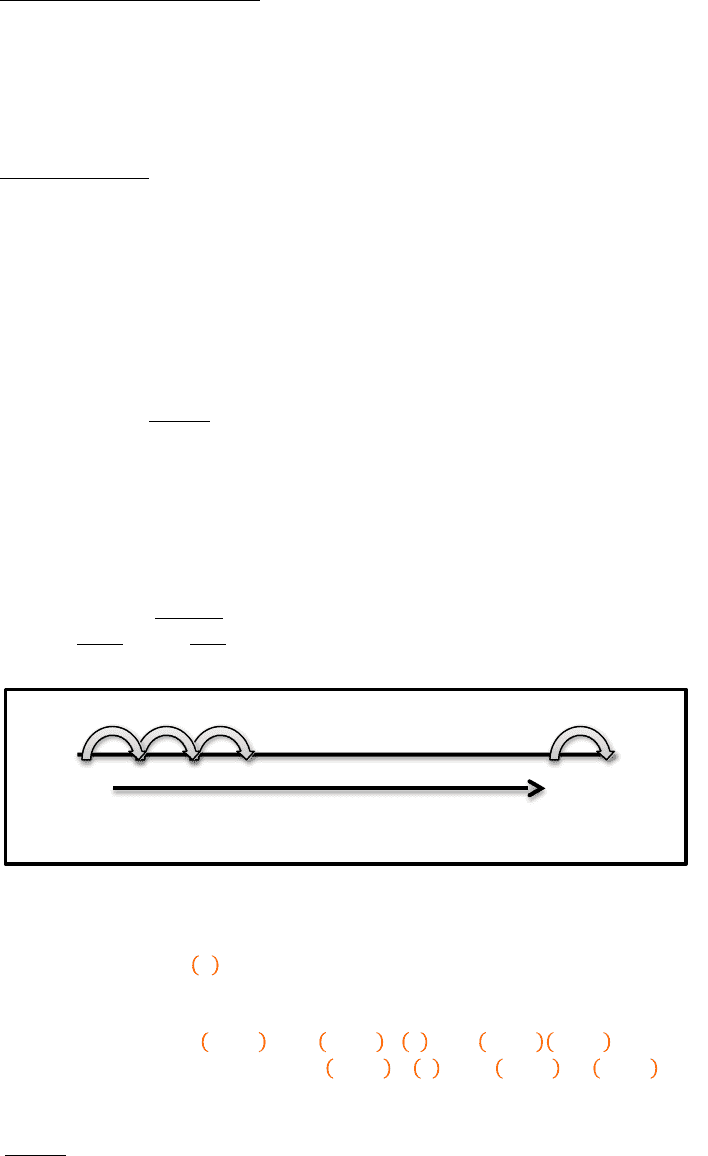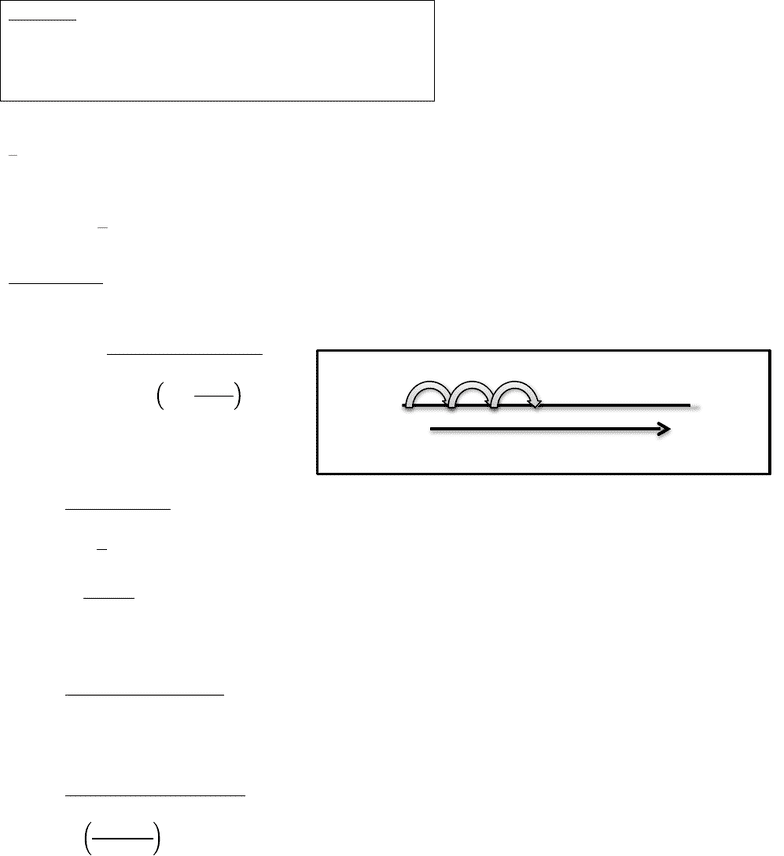Study Guides (390,000)
CA (150,000)
UTSC (10,000)
Mathematics (1,000)
MATA32H3 (100)
Midterm

# MATA32H3 Midterm: Term Test 1 - Fall 2017Premium

Department
Mathematics
Course Code
MATA32H3
Professor
Raymond Grinnell
Study Guide
Midterm

This preview shows pages 1-3. to view the full 37 pages of the document.
University of Toronto
Scarborough
MATA32H3
Calculus for Management I
Fall 2017
Term Test 1
Exam Guide

Only pages 1-3 are available for preview. Some parts have been intentionally blurred.

Subscribers OnlyCompound Interest
Compound Interest
Deals with money, time and interest
1 key equation:
𝑆=𝑃(1+𝑟)!
P ! Principle
Money invested now
r ! Periodic interest rate
Interest paid per compounding period
Stated in percentage or annual rate
oNOTE: Must convert percentage into a decimal
n ! Number of compounding periods
Counts number of times interest is paid on investment
S ! Compound Amount
Amount of money we have at the end
Interest is paid exactly at the end of compounding periods
Only at the end of compounds
**For one compound period, the principle (P) plus the interest on that
1st Compound ! 𝑃1+Pr =!𝑃!(1+𝑟)
2nd Compound ! 𝑃!1+𝑟+!𝑃!1+𝑟𝑟=𝑃!1+𝑟1+𝑟=𝑃!(1+𝑟)!
3rd Compound ! 𝑃!(1+𝑟)!+!𝑃!1+𝑟!𝑟=!𝑃!1+𝑟!1+𝑟=𝑃!(1+𝑟)!
4th Compound ! 𝑃!(1+𝑟)!
NOTE: IN APPLICATIONS
We often have APR ‘a(Annual Percentage Rate) and frequency ‘k’ of
compounding; and
oHow often interest is paid in a year
A time ‘t’ period
!
Khl
Compounding periods
End Start
Fixed Period of time
n!
P \$
(Principle!+!Interest!on!Principle)!

Only pages 1-3 are available for preview. Some parts have been intentionally blurred.

Subscribers OnlyLegend:
a ! APR for period as follows
k ! Compounding frequency per year (annually)
t ! Number of years for a particular period
We have that
!
!= r and 𝑛=𝑘𝑡
Thus,
𝑆=𝑃!(1+!
𝑎
𝑘)!!
Example 1: Consider the following “investment scheme” invest \$10,000 at 3.05% APR
compounding monthly for a period of 5 years.
a) Find compound amount
𝑆=10,000!1+!.!"!#
!"
!"
11,645.17
b) Periodic rate (interest at the end of each month)
𝑟=
𝑎
𝑘
=
0.0305
12
0.002542!(0.2542%)
c) Compound Interest
Difference between beginning amount and end amount
𝑆𝑃
1,645.17
Return due to interest
=
1645.17
10000
100
16.4517%
(Over 5 years accumulated)
d) How long does it take to earn \$15,000
** Express terms in units of compounding (i.e. from this example, months)**
5 years
\$10,000
\$11,645.17 X 60